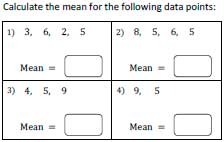Average or Mean Worksheets

Mean worksheets in this page contain finding arithmetic mean for bunch of whole numbers, calculating mean in practical units, finding average for the data points in decimals, finding missing item and more.

Average Worksheets - General

Average or arithmetic mean worksheets in this section have data values in whole numbers.Mean Worksheets: Easy Level

Simple mean worksheets for beginners. It includes average of 2, 3 or 4 numbers with various difficulty levels.

Numbers from 1 to 9:

Numbers from 1 to 99:

Mean Worksheets: Moderate Level

These worksheets include average of 4, 5 or 6 data points.

Numbers from 1 to 9:

Numbers from 1 to 99:

Mean Worksheets: Difficult Level

These worksheets include more than 6 data points.

Numbers from 1 to 99:

Average with Practical Units

Instead of mere numbers, these mean worksheets include practical units used in our day to day life.

Worksheets with Same Units: Easy

Find the mean of the numbers and write down the same unit after calculation. The question may have 3, 4 or 5 numbers.

Practical Unit 2

Worksheets with Different Units: Challenge

Convert the units as necessary before you start calculating mean. For an example, if the data contains 4 numbers out of which 2 numbers in grams and 2 in kilograms, but the mean is required in grams, then change kilogram into gram and calculate the mean.

Challenge 2

Mean Worksheets - Decimal Numbers

Mean worksheets contain data points in decimals. Easy worksheets include 3 or 4 decimals, 'moderate' includes 4 to 6 decimal points and 'difficult' includes 7 to 12 data points.

Decimal Data: Easy

Calculate Mean 2

Decimal Data: Moderate

Calculate Mean 3

Calculate Mean 4

Decimal Data: Difficult

Calculate Mean 6

Missing Number - Challenging Problems

Find the missing number using data points and mean. Apply Algebra method of solving equations to easily solve these worksheets.2 data points

Missing Number 2

3 or 4 data points

Missing Number 1

Missing Number 2

5 or 6 data points

Missing Number 2

Identify the Decimal

Missing Decimal: Easy

Missing Decimal: Moderate

Mean Absolute Deviation Worksheets

Employ our mean absolute deviation worksheets to find the mean and mean absolute deviations up to 6 and 10 data sets involving whole numbers and decimals. Compare two data sets as well.

Mean Absolute Deviation (21 Worksheets)

The entire collection of worksheets on average or mean can be downloaded in a jiffy!

Related Worksheets